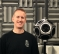## Upward Compressor Soft Knee EquationStarted by 3 years ago4 replieslatest reply 3 years ago767 views

I am working on a Python function to perform audio dynamic range compression using an upward compressor (https://en.wikipedia.org/wiki/Dynamic_range_compre...). I am trying to implement soft knee controls but am having trouble figuring out the correct equation (similar to equations for downward compression shown here https://dsp.stackexchange.com/questions/28548/diff...). If anyone knows how to calculate the upward compression soft knee or has any references on the subject please let me know, thanks!

[ - ]Use almost the same equations. Just reverse the first and last ranges (so that the last range has slope 1) and in the middle range reverse the sign of the 2nd term and that of the W/2 term. (Incidentally, in the dsp.stackexchange answer the sign of W/2 looks wrong to me.)

Y(J)S

[ - ]Thank you! I figured it was straightforward I was just overthinking it.

For clarity, the Upward Compressor w/Soft Knee static characteristic gain equations are:

g_sc[n] = x_dB[n]    if x_dB[n] > T + W/2

= x_dB[n] - [(1/R-1)(x_dB[n]-T-W/2)^2] /[2W]                   if (T-W/2) < x_dB[n] < (T+W/2)

= T + (x_dB[n] - T) / R        if x_dB[n] < T - W/2

[ - ]almost

the middle equation should have + (1/R - 1)

here's my MATLAB :

x = 0:0.001:1 ;

T = 0.5 ;  % kick-in point (sample 501)

W = 0.2 ;  % soft knee width (10 samples)

W2 = W/2 ;

R = 5 ;    % compression ratio

f = -(1/R - 1)/(2*W) ;

% hard knee downward compression

yhd = zeros(1, 1001) ;

yhd(1:500) = x(1:500) ;

yhd(501:end) = T + (x(501:end)-T)/R ;

% hard knee upward compression

yhu = zeros(1, 1001) ;

yhu(1:500) = T + (x(1:500)-T)/R ;

yhu(501:end) = x(501:end) ;

% soft knee upward compression

ysu = zeros(1, 1001) ;

ysu(1:400) = T + (x(1:400)-T)/R ;

ysu(401:600) = x(401:600) + f * ( x(401:600) - T - W/2 ).^2 ;

ysu(601:end) = x(601:end) ;

figure

plot(x,yhu,'k', x,ysu,'r') ;

title('upward compression')

axis([0,1,0,1])

% soft knee downward compression

ysd = zeros(1, 1001) ;

ysd(1:400) = x(1:400) ;

ysd(401:600) = x(401:600) - f * ( x(401:600) - T + W/2 ).^2 ;

ysd(601:end) = T + (x(601:end)-T)/R ;

figure

plot(x,yhd,'k', x,ysd,'b') ;

title('downward compression')

axis([0,1,0,1])

[ - ]In your code, for the upward soft knee portion you have

ysu(401:600) = x(401:600) + f * ( x(401:600) - T - W/2 ).^2 ;

and f is defined as

f = -(1/R - 1)/(2*W) ;

So when you plug f into that expression, it ends up being -(1/R - 1). My matlab code based on my posted equations provides the same results. The +(1/R-1) is then for downward compression, which your code also indicates.